## (TD) 4.7 Generating Capacitor Charge and Discharge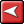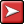## (TD) 4.7 Generating Capacitor Charge and DischargeBoth Waveform Block Equations and Label Equations can be used to draw capacitor charge and discharge waveforms. These  functions have three required parameters - amplitude, RC constant, and duration - that are used to generate the waveform.

For Label equations, there is also an optional  fourth parameter that can specify the number of points to use when drawing the waveform. For Waveform Block Equations, the number of points is determined by durationT/deltaT.

Capacitor Charging Waveforms with State Label Equations

The CapCharge function generates the waveform for a charging capacitor. The waveform will start at zero and gradually rise (using the RC value) to the amplitude at the end of duration specified.

 •Add a new signal to the diagram and double click on it to open the Signal Properties dialog. Name the signal Capacitor_Waveform and set the Size ratio to 2. •Enter CapCharge(5, 10, 50) in the Label Eqn box either by typing or by using the equation flyout. The  parameters are amplitude of 5, RC constant of 10, and duration of 50ns (assuming the ns is the display time unit).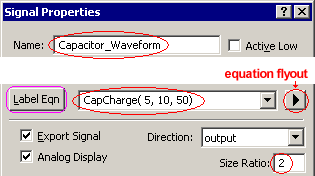•Click Label Eqn to generate the capacitor charging waveform. The timing diagram editor  will automatically change the radix of the signal to real, because the generator uses floating point numbers to model the waveform.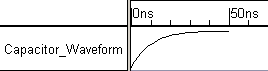Capacitor Discharge Waveforms with State Label Equations

The CapDischarge function performs the inverse of the CapCharge function. The waveform starts at the maximum amplitude and it slowly declines based on the RC provided over the duration until it reaches the lowest point.

 •Enter CapDischarge(5,10,50) in the Label Eqn box by typing or by using the equation flyout. This generates a capacitor discharge waveform that starts at an amplitude of 5, and discharges at a rate controled by an RC value of 10, and a duration of 50ns (assuming that the display time unit is ns).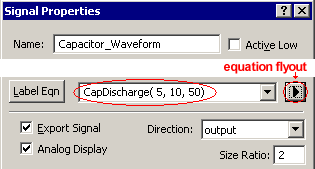•Press the Label Eqn button to generate the waveform.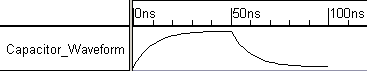The Capacitor label equations append to the end of the waveform, so you can also draw part of the waveform and then append a capacitor waveform to the drawn signal.